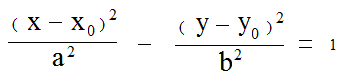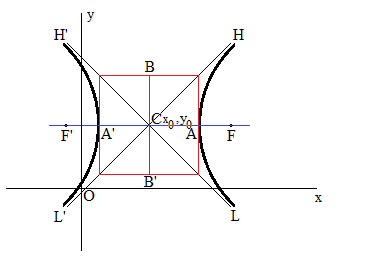## Hyperbolic equation Calculator

 x0 :y0 : a  :b   :
 Hyperbola Focus F: Hyperbola Focus F': Asymptotes H'L : Asymptotes L'H : Hyperbola Eccentricity :

In mathematics, a plural hyperbolas or hyperbola or hyperbolae is a type of smooth curve, it has two pieces, named as components or branches, they resemble two infinite bows and are mirrow images of each other. the hyperbola lying in a plane.

### Hyperbola formula:### Hyperbola graph:Hyperbola equation and graph with center C(x0, y0) and major axis parallel to x axis. If the major axis is parallel to the y axis, interchange x and y during the calculation.

### Hyperbola calculator equations:

Hyperbola Focus F X Coordinate  =  x0 + √(a2 + b2)

Hyperbola Focus F Y Coordinate  =  y0

Hyperbola Focus F' X Coordinate  =  x0 - √(a2 + b2)

Hyperbola Focus F' Y Coordinate  =  y0

Asymptotes H'L: y=(b/a)x + y0 - (b/a)x0

Asymptotes LH': y=(-b/a)x + y0 + (b/a)x0

Hyperbola Eccentricity  =  √(a2 + b2) / a

Thinkcalculator.com provides you helpful and handy calculator resources.